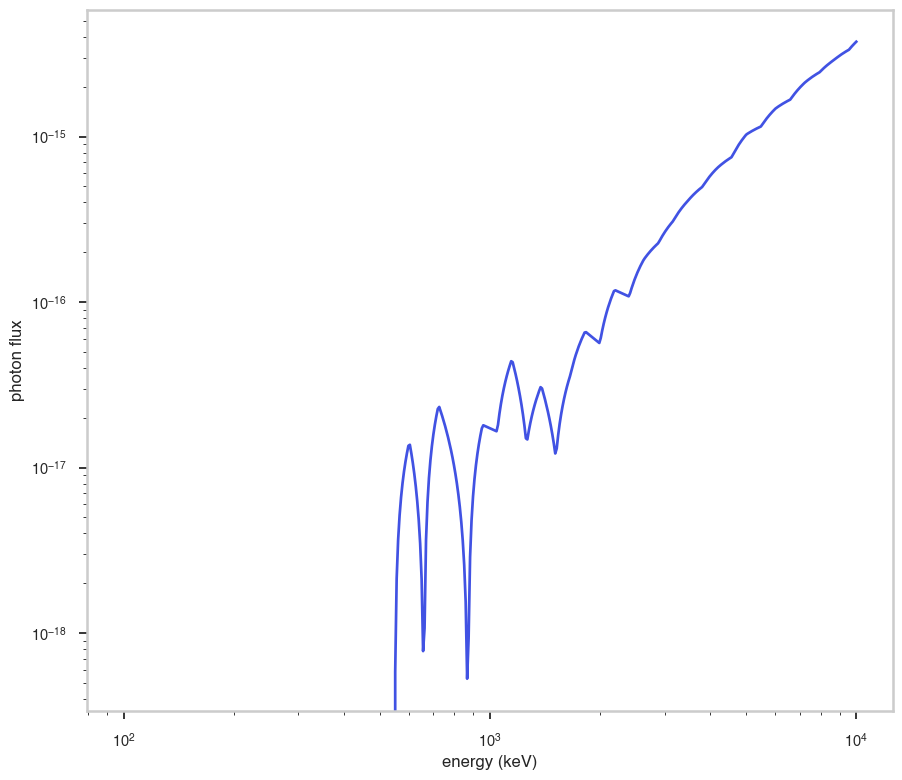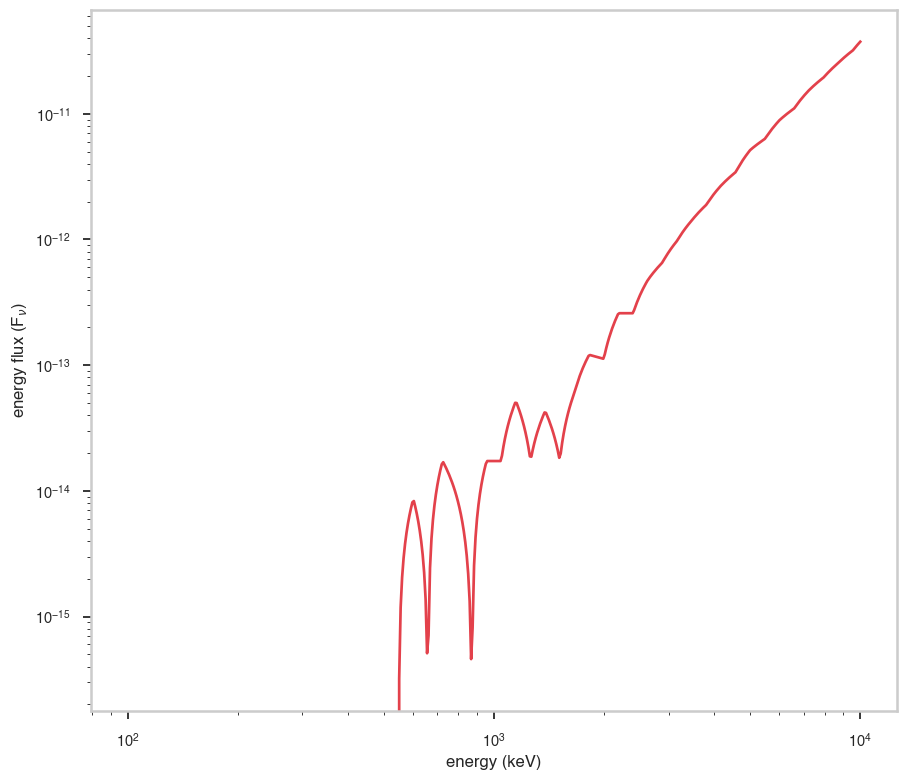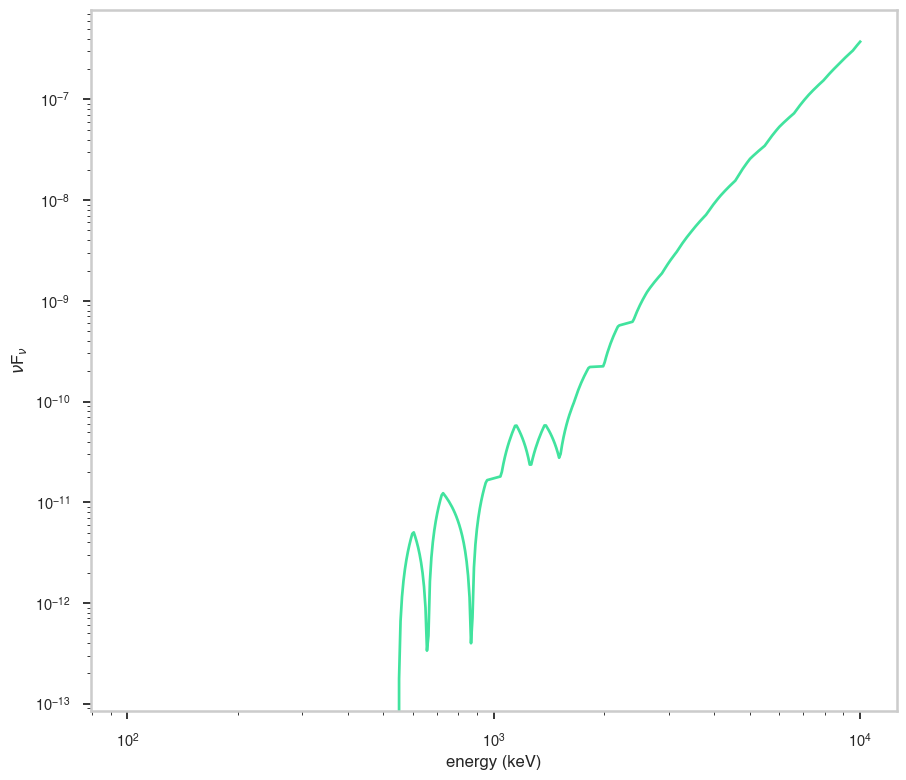# DMSpectra

:

# Parameters
func_name = "DMSpectra"
wide_energy_range = True
x_scale = "log"
y_scale = "log"
linear_range = False


## Description

:

func.display()

• description: Class that evaluates the spectrum for a DM particle of a given mass, channel, cross section, and J-factor. Combines Pythia-based tables from both Fermi (2 GeV < m_DM < 10 TeV) and HAWC (10 TeV < m_dm < 1 PeV) The parameterization is given by F(x) = 1 / (8 * pi) * (1/mass^2) * sigmav * J * dN/dE(E,mass,i) Note that this class assumes that mass and J-factor are provided in units of GeV and GeV^2 cm^-5
• formula: 
• parameters:
• mass:
• value: 10.0
• desc: DM mass (GeV)
• min_value: None
• max_value: None
• unit:
• is_normalization: False
• delta: 1.0
• free: False
• channel:
• value: 4.0
• desc: DM annihilation channel
• min_value: None
• max_value: None
• unit:
• is_normalization: False
• delta: 0.4
• free: False
• sigmav:
• value: 1e-26
• desc: DM annihilation cross section (cm^3/s)
• min_value: None
• max_value: None
• unit:
• is_normalization: False
• delta: 1e-27
• free: True
• J:
• value: 1e+20
• desc: Target total J-factor (GeV^2 cm^-5)
• min_value: None
• max_value: None
• unit:
• is_normalization: False
• delta: 1e+19
• free: False

## Shape

The shape of the function.

If this is not a photon model but a prior or linear function then ignore the units as these docs are auto-generated

:

fig, ax = plt.subplots()

ax.plot(energy_grid, func(energy_grid), color=blue)

ax.set_xlabel("energy (keV)")
ax.set_ylabel("photon flux")
ax.set_xscale(x_scale)
ax.set_yscale(y_scale)## F$$_{\nu}$$

The F$$_{\nu}$$ shape of the photon model if this is not a photon model, please ignore this auto-generated plot

:

fig, ax = plt.subplots()

ax.plot(energy_grid, energy_grid * func(energy_grid), red)

ax.set_xlabel("energy (keV)")
ax.set_ylabel(r"energy flux (F$_{\nu}$)")
ax.set_xscale(x_scale)
ax.set_yscale(y_scale)## $$\nu$$F$$_{\nu}$$

The $$\nu$$F$$_{\nu}$$ shape of the photon model if this is not a photon model, please ignore this auto-generated plot

:

fig, ax = plt.subplots()

ax.plot(energy_grid, energy_grid**2 * func(energy_grid), color=green)

ax.set_xlabel("energy (keV)")
ax.set_ylabel(r"$\nu$F$_{\nu}$")
ax.set_xscale(x_scale)
ax.set_yscale(y_scale)